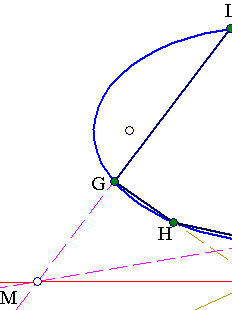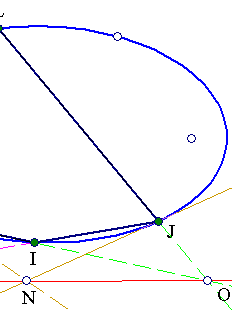##1. Pascal's theorem

The interesection points of the opposite sides of a hexagon inscribed in a conic are collinear (on
a line named a Pascal line of the hexagon (red line in the figure)).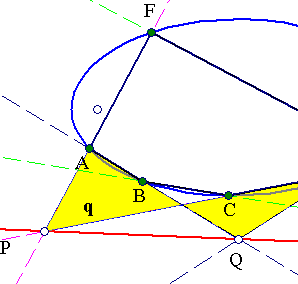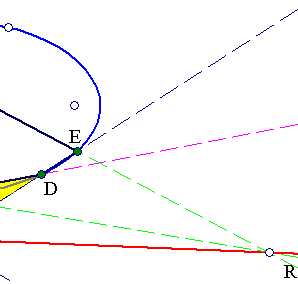A proof can be given by transferring verbatim the arguments for the case in which the conic is
a circle, to be found in Pascal.html .
 Another line of proof is to consider a projectivity F mapping the conic onto the circle and use
again the special proof for the circle.
 A third proof I like is the ingenious one given by Salmon [SalmonConics,p.221]:
Denote by (AB)=0 the equation of the line joining points A and B. Since the conic passes through
points {A,B,C,D} it can be represented in the form
For the same reason i.e. because it passes through {D,E,F,A} it can be represented in the form
Equating these expressions we obtain
Left hand side represents a conic circumcscribing quadrilateral (q) with sides {AB,DE,CD,AF} i.e.
passing through points {A,D,P,Q}. The right hand side is a product of lines one of which is the
diagonal (AD)=0 of (q). Hence the other factor ((BC)-(EF))=0 represents the other diagonal
(PQ)=0 of (q).
But from its form follows that it passes also through the intersection point of lines (BC)=0 and
(EF)=0, which is R.
 A fourth ingenious proof is due to [ChaslesApercu, p. 149], see also [Cayley, v1 p.25] and relates
to cubic curves and the following simple fact for them:
If a cubic c3 passes through eight out of the nein intersection points of two cubics
c1, c2 then it passes also through the neinth
.
The proof of Pascal's theorem follows by applying this theorem to the two (degenerate) cubics c1, c2
consisting from lines c1=AB*CD*EF and c2=BC*DE*FA (meant is the product of the lines).
The two cubics intersect at nine points: the 6 vertices of the hexagon and the 3 intersections of opposite
sides. Consider then the cubic c3 consisting of the conic and the line passing through two out of the
three intersections of opposite sides, say points P and Q. Then, by the aforementioned theorem this cubic
will pass also from the ninth intersection point which is R necessarily lying on line PQ.

##2. Construction of more than 5 points

A conic is completely defined by giving five points. Pascal's theorem allows the construction of
the 6th point on any line passing through one of these five points. Thus through this theorem,
starting by five arbitrary points we can construct arbitrary many points on the conic defined by
these five points.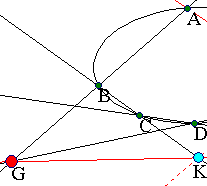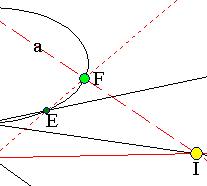Given {A,B,C,D,E} draw a (variable) line a through A and find its intersection I with CD. Let G
be the intersection of lines (AB, DE). Draw line GI and find its intersection K with line BC. The
sixth point F on line a must be on line KE according to Pascal.

##3. Pascal for degenerate hexagons

Notice that Pascal's theorem is valid also in the case of degenerate hexagons, i.e. when some
consecutive vertices coincide, a case where the line joining two points, must be replaced with the
tangent at the (double) point. This is the case with the figure below, where at J we take the
tangent to the conic.
The figure gives also a recipe for the geometric construction of the tangent at a point J of the
conic, using points on the conic, lines and their intersections:
We define four other points I, H, G and L and join as shown. All dotted lines are known, and
points M, O are immediately constructed. Then N is determined as intersection point of MO and
GH. From this follows the construction of the tangent, by joining N and J.
See the references below for some other aspects of Pascal's theorem.